## Excel XIRR Function

This post will guide you how to use Excel XIRR function with syntax and examples in Microsoft excel.

### Description

The Excel XIRR function returns the internal rate of return for a series of cash flows that is not necessarily periodic. And if you want to get the internal rate of return for a series of periodic cash flows, you can use the IRR function in Excel.

The XIRR function is a build-in function in Microsoft Excel and it is categorized as a Financial Function.

The XIRR function is available in Excel 2016, Excel 2013, Excel 2010, Excel 2007, Excel 2011 for Mac.

### Syntax

The syntax of the XIRR function is as below:

`= XIRR (values, dates, [guess])`

Where the XIRR function arguments are:

• Values -This is a required argument. An array or cell reference that contain numbers for which you want to calculate the internal rate of return. And the values must include at least one positive value and one negative value.
• Dates -This is a required argument. A series of dates that corresponds to the cash flow payments.
• Guess – This is an optional argument. An initial guess at the internal rate of return. If it is omitted, and it will be set as 0.1 or 10%.

Note:

• The values arguments must contain at least one positive value and one negative cash flow value, or the XIRR function will return the #NUM! Error.
• If you provide an invalid date in the date argument, the XIRR function will return the #NUM! Error.

### Excel XIRR Function Examples

The below examples will show you how to use Excel XIRR Function to calculate the internal rate of return for a series of cash flows.

#1 to get the internal rate of return, using the following formula:

`=XIRR(A2:A5,B2:B5)`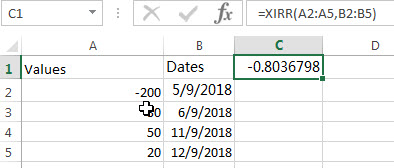### Related Functions

• Excel IRR Function
The Excel IRR function returns the internal rate of return for a series of cash flows and the cash flows must be occurred at regular intervals (monthly or annually).The syntax of the IRR function is as below:=IRR(values, [guess])…

### Related Examples

• Calculate Stock Rate of Return
You can try to calculate the rate of return by manually, or you use an Excel formula to achieve the result. The best way to calculate your rate of return is to use the EXCEL XIRR function,…

## Excel VDB Function

This post will guide you how to use Excel VDB function with syntax and examples in Microsoft excel.

### Description

The Excel VDB function calculates the depreciation of an asset for a specified period based on the double declining balance method. And it will return a numeric value.

The VDB function is a build-in function in Microsoft Excel and it is categorized as a Financial Function.

The VDB function is available in Excel 2016, Excel 2013, Excel 2010, Excel 2007, Excel 2011 for Mac.

### Syntax

The syntax of the VDB function is as below:

`= VDB (cost, salvage, life, start_period, end_period, [factor], [no_switch])`

Where the VDB function arguments are:

• Cost -This is a required argument. The initial cost of the asset.
• Salvage -This is a required argument. The value of the asset at the end of the depreciation.
• Life -This is a required argument. The number of periods over which the asset is to be depreciated.
• Start_period -This is a required argument. The starting period for which you want to calculate the depreciation.
• End_period -This is a required argument. The ending period for which you want to calculate the depreication.
• Factor -This is an optional argument. It is specified the rate of depreciation.
• No_switch -This is a required argument. A logical value specifying whether to switch to straight-line depreciation when depreciation is greater than the declining balance calculation. And it can be either a value of TRUE or FALSE.
 TRUE Using declining balance method of depreciation. FALSE Using the straight-line depreciation method when the straight-line depreciation is greater than the declining balance depreciation amount.

### Excel VDB Function Examples

The below examples will show you how to use Excel VDB Function to calculate the straight line depreciation of an asset for one period.

#1 to get the depreciation between the 7th and 12th month for an asset that cost 3000, with a salvage value of 500, and the useful life of the asset is 5, using the following formula:

`=VDB(B1,B2,B3*12,7,12)`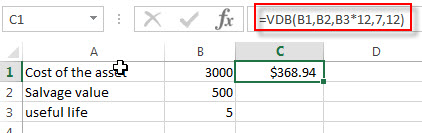### Related Functions

• Excel SLN Function
The Excel SLN function calculates the depreciation of an asset for one period based on the straight line depreciation. And it will return a numeric value.The syntax of the SLN function is as below:= SLN (cost, salvage, life)…
• Excel SYD Function
The Excel SYD function calculates the sum-of-years’digits depreciation of an asset for a specified period. And it will return a numeric value.The syntax of the SYD function is as below:=SYD(cost, salvage, life, per)…

## Excel SYD Function

This post will guide you how to use Excel SYD function with syntax and examples in Microsoft excel.

### Description

The Excel SYD function calculates the sum-of-years’digits depreciation of an asset for a specified period. And it will return a numeric value.

The SYD function is a build-in function in Microsoft Excel and it is categorized as a Financial Function.

The SYD function is available in Excel 2016, Excel 2013, Excel 2010, Excel 2007, Excel 2011 for Mac.

### Syntax

The syntax of the SYD function is as below:

`=SYD(cost, salvage, life, per)`

Where the SYD function arguments are:

• Cost -This is a required argument. The initial cost of the asset.
• Salvage -This is a required argument. The value of the asset at the end of the depreciation.
• Life -This is a required argument. The number of periods over which the asset is to be depreciated.
• Per -This is a required argument. The period that you want to calculate the depreciation for.

### Excel SYD Function Examples

The below examples will show you how to use Excel SYD Function to calculate the sum-of-years’digits depreciation for a period in the lifetime of an asset.

#1 to get the depreciation for the first year for an asset that cost 3000, with a salvage value of 500, and the useful life of the asset is 5, using the following formula:

`=SYD(B1,B2,B3,1) `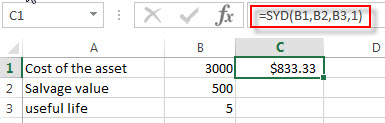### Related Functions

• Excel SLN Function
The Excel SLN function calculates the depreciation of an asset for one period based on the straight line depreciation. And it will return a numeric value.The syntax of the SLN function is as below:= SLN (cost, salvage, life)…
• Excel VDB Function
The Excel VDB function calculates the depreciation of an asset for a specified period based on the double declining balance method. And it will return a numeric value.The syntax of the VDB function is as below:= VDB (cost, salvage, life, start_period, end_period, [factor], [no_switch])…

## Excel SLN Function

This post will guide you how to use Excel SLN function with syntax and examples in Microsoft excel.

### Description

The Excel SLN function calculates the depreciation of an asset for one period based on the straight line depreciation. And it will return a numeric value.

The SLN function is a build-in function in Microsoft Excel and it is categorized as a Financial Function.

The SLN function is available in Excel 2016, Excel 2013, Excel 2010, Excel 2007, Excel 2011 for Mac.

### Syntax

The syntax of the SLN function is as below:

`= SLN (cost, salvage, life)`

Where the SLN function arguments are:

• Cost -This is a required argument. The initial cost of the asset.
• Salvage -This is a required argument. The value of the asset at the end of the depreciation.
• Life -This is a required argument. The number of periods over which the asset is to be depreciated.

### Excel SLN Function Examples

The below examples will show you how to use Excel SLN Function to calculate the straight line depreciation of an asset for one period.

#1 to get the depreciation for an asset that cost 3000, with a salvage value of 500, and the useful life of the asset is 5, using the following formula:

= SLN (B1,B2,B3,B4)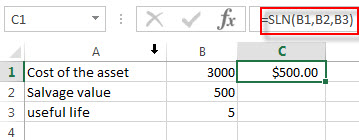### Related Functions

• Excel SYD Function
The Excel SYD function calculates the sum-of-years’digits depreciation of an asset for a specified period. And it will return a numeric value.The syntax of the SYD function is as below:=SYD(cost, salvage, life, per)…
• Excel VDB Function
The Excel VDB function calculates the depreciation of an asset for a specified period based on the double declining balance method. And it will return a numeric value.The syntax of the VDB function is as below:= VDB (cost, salvage, life, start_period, end_period, [factor], [no_switch])…

## Excel PV Function

This post will guide you how to use Excel PV function with syntax and examples in Microsoft excel.

### Description

The Excel PV function returns the present value of a loan or investment based on constant payments and a constant interest rate. So you can use the PV function to get the present value based on a series of future payments.

The PV function is a build-in function in Microsoft Excel and it is categorized as a Financial Function.

The PV function is available in Excel 2016, Excel 2013, Excel 2010, Excel 2007, Excel 2011 for Mac.

### Syntax

The syntax of the PV function is as below:

`= PV(rate,nper,pmt,[fv],[type])`

Where the PV function arguments are:

• Rate -This is a required argument. The interest rate per period.
• nPer -This is a required argument. The total number of payments periods in an annuity.
• Pmt – This is a required argument. The amount of the payment made each period.
• Fv – This is an optional argument. The future value or a cash balance you want to attain after the last payment is made. If fv is omitted, it is assumed to be 0 (zero), that is, the future value of a loan is 0.
• Type – This is an optional argument. The number 0 (zero) or 1 and indicates when payments are due.
 Set type equal to If payments are due 0 or omitted At the end of the period 1 At the beginning of the period

Note:

• Make sure that you are consistent about the units you use for specifying rate and nper. If you make monthly payments on a four-year loan at an annual interest rate of 12 percent, use 12%/12 for rate and 4*12 for nper. If you make annual payments on the same loan, use 12 percent for rate and 4 for nper.

### Excel PV Function Examples

The below examples will show you how to use Excel PV Function to calculate the present value of an investment.

#1 to get the present value of an annuity with the terms in A2:A4, using the following formula:

`=PV(B1,B2,B3,B4)`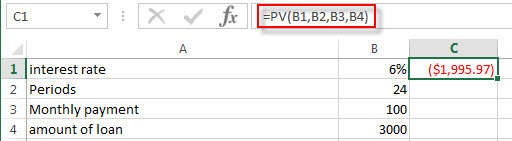### Related Functions

• Excel FV Function
The Excel FV function used to calculate the future value of an investment based on a constant interest rate. The syntax of the FV function is as below:=FV(rate,nper,pmt,[pv],[type])…
• Excel IPMT Function
The Excel IPMT function used to calculate the interest payment for an investment based on a constant payment schedule and a constant interest rage.The syntax of the IPMT function is as below:= IPMT (rate, per, nper, pv, [fv], [type])…
• Excel IRR Function
The Excel IRR function returns the internal rate of return for a series of cash flows and the cash flows must be occurred at regular intervals (monthly or annually).The syntax of the IRR function is as below:=IRR(values, [guess])…
• Excel ISPMT Function
The Excel ISPMT function used to calculate the interest paid during a specific period of an investment.The syntax of the ISPMT function is as below:= ISPMT (rate, per, nper, pv)…
• Excel MIRR Function
The Excel MIRR function returns the modified internal rate of return for a series of cash flows and the cash flows must be occurred at regular intervals (monthly or annually). The syntax of the MIRR function is as below:=MIRR(values, finance_rate,reinvest_rate)…
Excel NPER Function
The Excel NPER function returns the number of periods for an investment or loan based on periodic payment amount and a constant interest rate.The syntax of the NPER function is as below:= NPER (rate, pmt, pv, [fv], [type])…
• Excel NPV Function
The Excel NPV function returns the net present value of an investment by using a discount rate and a series of future cash flows (future payments and income).The syntax of the NPV function is as below:= NPV (rate, Value1,[value2],…)…
• Excel PMT Function
The Excel PMT function returns the payment amount for a loan or investment based on constant payments and a constant interest rate.The syntax of the PMT function is as below:= PMT(rate, nper, pv,[fv],[type])…
• Excel PPMT Function
The Excel PPMT function returns the payment amount on the principal for a given period for a loan or investment based on constant payments and a constant interest rate. The syntax of the PPMT function is as below:=PPMT(rate, per,nper, pv,[fv],[type])…
• Excel RATE Function
The Excel RATE function returns the interest rate per payment period of an annuity.The syntax of the RATE function is as below:=RATE(nper, pmt,pv,[fv],[type],[guess])…

## Excel RATE Function

This post will guide you how to use Excel RATE function with syntax and examples in Microsoft excel.

### Description

The Excel RATE function returns the interest rate per payment period of an annuity.

The RATE function is a build-in function in Microsoft Excel and it is categorized as a Financial Function.

The RATE function is available in Excel 2016, Excel 2013, Excel 2010, Excel 2007, Excel 2011 for Mac.

### Syntax

The syntax of the RATE function is as below:

`=RATE(nper, pmt,pv,[fv],[type],[guess])`

Where the RATE function arguments are:

• nPer -This is a required argument. The total number of payments periods in an annuity.
• Pmt – This is a required argument. The amount of the payment made each period.
• Pv – This is a required argument. The present value of the payments.
• Fv – This is an optional argument. The future value or a cash balance you want to attain after the last payment is made. If fv is omitted, it is assumed to be 0 (zero), that is, the future value of a loan is 0.
• Type – This is an optional argument. The number 0 (zero) or 1 and indicates when payments are due.
 Set type equal to If payments are due 0 or omitted At the end of the period 1 At the beginning of the period
• Guess – This is an optional argument. An initial guess at the internal rate of return. if it is omitted, and it will be set as 0.1 or 10%.

Note:

• Make sure that you are consistent about the units you use for specifying rate and nper. If you make monthly payments on a four-year loan at an annual interest rate of 12 percent, use 12%/12 for rate and 4*12 for nper. If you make annual payments on the same loan, use 12 percent for rate and 4 for nper.

### Excel RATE Function Examples

The below examples will show you how to use Excel RATE Function to calculate the interest rate per period of an annuity.

#1 to get the monthly rate of the loan, using the following formula:

`=RATE(B1*12,B2,B3)`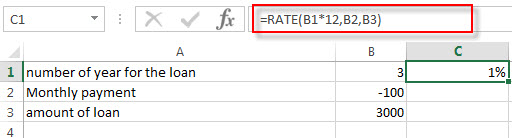### Related Functions

• Excel FV Function
The Excel FV function used to calculate the future value of an investment based on a constant interest rate. The syntax of the FV function is as below:=FV(rate,nper,pmt,[pv],[type])…
• Excel IPMT Function
The Excel IPMT function used to calculate the interest payment for an investment based on a constant payment schedule and a constant interest rage.The syntax of the IPMT function is as below:= IPMT (rate, per, nper, pv, [fv], [type])…
• Excel IRR Function
The Excel IRR function returns the internal rate of return for a series of cash flows and the cash flows must be occurred at regular intervals (monthly or annually).The syntax of the IRR function is as below:=IRR(values, [guess])…
• Excel ISPMT Function
The Excel ISPMT function used to calculate the interest paid during a specific period of an investment.The syntax of the ISPMT function is as below:= ISPMT (rate, per, nper, pv)…
• Excel MIRR Function
The Excel MIRR function returns the modified internal rate of return for a series of cash flows and the cash flows must be occurred at regular intervals (monthly or annually). The syntax of the MIRR function is as below:=MIRR(values, finance_rate,reinvest_rate)…
Excel NPER Function
The Excel NPER function returns the number of periods for an investment or loan based on periodic payment amount and a constant interest rate.The syntax of the NPER function is as below:= NPER (rate, pmt, pv, [fv], [type])…
• Excel NPV Function
The Excel NPV function returns the net present value of an investment by using a discount rate and a series of future cash flows (future payments and income).The syntax of the NPV function is as below:= NPV (rate, Value1,[value2],…)…
• Excel PMT Function
The Excel PMT function returns the payment amount for a loan or investment based on constant payments and a constant interest rate.The syntax of the PMT function is as below:= PMT(rate, nper, pv,[fv],[type])…
• Excel PPMT Function
The Excel PPMT function returns the payment amount on the principal for a given period for a loan or investment based on constant payments and a constant interest rate. The syntax of the PPMT function is as below:=PPMT(rate, per,nper, pv,[fv],[type])…
• Excel PV Function
The Excel PV function returns the present value of a loan or investment based on constant payments and a constant interest rate. So you can use the PV function to get the present value based on a series of future payments.The syntax of the PV function is as below:= PV(rate,nper,pmt,[fv],[type])…

## Excel PPMT Function

This post will guide you how to use Excel PPMT function with syntax and examples in Microsoft excel.

### Description

The Excel PPMT function returns the payment amount on the principal for a given period for a loan or investment based on constant payments and a constant interest rate. So you can use PPMT function to get the principal amount of a payment for a specified period.

The PPMT function is a build-in function in Microsoft Excel and it is categorized as a Financial Function.

The PPMT function is available in Excel 2016, Excel 2013, Excel 2010, Excel 2007, Excel 2011 for Mac.

### Syntax

The syntax of the PPMT function is as below:

`=PPMT(rate, per,nper, pv,[fv],[type])`

Where the PPMT function arguments are:

• Rate -This is a required argument. The interest rate for the loan.
• per -This is a required argument. The period for which the payment on the principal is to be calculated. And it must be an integer number between 1 and nper value.
• nPer -This is a required argument. The total number of payments for the loan.
• Pv – This is a required argument. The present value of the payments.
• Fv – This is an optional argument. The future value or a cash balance you want to attain after the last payment is made. If fv is omitted, it is assumed to be 0 (zero), that is, the future value of a loan is 0.
• Type – This is an optional argument. The number 0 (zero) or 1 and indicates when payments are due.
 Set type equal to If payments are due 0 or omitted At the end of the period 1 At the beginning of the period

Note:

• Make sure that you are consistent about the units you use for specifying rate and nper. If you make monthly payments on a four-year loan at an annual interest rate of 12 percent, use 12%/12 for rate and 4*12 for nper. If you make annual payments on the same loan, use 12 percent for rate and 4 for nper.

### Excel PPMT Function Examples

The below examples will show you how to use Excel PPMT Function to calculate the principal payment amount for a loan based on an interest rate and a specified period.

#1 to get the principal payment for month 1 of the loan or investment, using the following formula:

`=PPMT(B1/12,1,B2*12,B3)`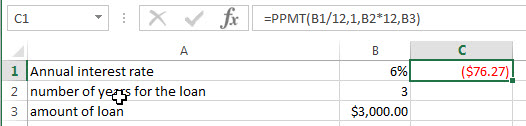### Related Functions

• Excel FV Function
The Excel FV function used to calculate the future value of an investment based on a constant interest rate. The syntax of the FV function is as below:=FV(rate,nper,pmt,[pv],[type])…
• Excel IPMT Function
The Excel IPMT function used to calculate the interest payment for an investment based on a constant payment schedule and a constant interest rage.The syntax of the IPMT function is as below:= IPMT (rate, per, nper, pv, [fv], [type])…
• Excel IRR Function
The Excel IRR function returns the internal rate of return for a series of cash flows and the cash flows must be occurred at regular intervals (monthly or annually).The syntax of the IRR function is as below:=IRR(values, [guess])…
• Excel ISPMT Function
The Excel ISPMT function used to calculate the interest paid during a specific period of an investment.The syntax of the ISPMT function is as below:= ISPMT (rate, per, nper, pv)…
• Excel MIRR Function
The Excel MIRR function returns the modified internal rate of return for a series of cash flows and the cash flows must be occurred at regular intervals (monthly or annually). The syntax of the MIRR function is as below:=MIRR(values, finance_rate,reinvest_rate)…
Excel NPER Function
The Excel NPER function returns the number of periods for an investment or loan based on periodic payment amount and a constant interest rate.The syntax of the NPER function is as below:= NPER (rate, pmt, pv, [fv], [type])…
• Excel NPV Function
The Excel NPV function returns the net present value of an investment by using a discount rate and a series of future cash flows (future payments and income).The syntax of the NPV function is as below:= NPV (rate, Value1,[value2],…)…
• Excel PMT Function
The Excel PMT function returns the payment amount for a loan or investment based on constant payments and a constant interest rate.The syntax of the PMT function is as below:= PMT(rate, nper, pv,[fv],[type])…
• Excel RATE Function
The Excel RATE function returns the interest rate per payment period of an annuity.The syntax of the RATE function is as below:=RATE(nper, pmt,pv,[fv],[type],[guess])…
• Excel PV Function
The Excel PV function returns the present value of a loan or investment based on constant payments and a constant interest rate. So you can use the PV function to get the present value based on a series of future payments.The syntax of the PV function is as below:= PV(rate,nper,pmt,[fv],[type])…

## Excel PMT Function

This post will guide you how to use Excel PMT function with syntax and examples in Microsoft excel.

### Description

The Excel PMT function returns the payment amount for a loan or investment based on constant payments and a constant interest rate.

The PMT function is a build-in function in Microsoft Excel and it is categorized as a Financial Function.

The PMT function is available in Excel 2016, Excel 2013, Excel 2010, Excel 2007, Excel 2011 for Mac.

### Syntax

The syntax of the PMT function is as below:

`= PMT(rate, nper, pv,[fv],[type])`

Where the PMT function arguments are:

• Rate -This is a required argument. The interest rate for the loan.
• nPer – This is a required argument. The total number of payments for the loan.
• Pv – This is a required argument. The present value of the payments.
• Fv – This is an optional argument. The future value or a cash balance you want to attain after the last payment is made. If fv is omitted, it is assumed to be 0 (zero), that is, the future value of a loan is 0.
• Type – This is an optional argument. The number 0 (zero) or 1 and indicates when payments are due.
 Set type equal to If payments are due 0 or omitted At the end of the period 1 At the beginning of the period

Note:

• The payment returned by PMT includes principal and interest but no taxes, reserve payments, or fees sometimes associated with loans.
• Make sure that you are consistent about the units you use for specifying rate and nper. If you make monthly payments on a four-year loan at an annual interest rate of 12 percent, use 12%/12 for rate and 4*12 for nper. If you make annual payments on the same loan, use 12 percent for rate and 4 for nper.

### Excel PMT Function Examples

The below examples will show you how to use Excel PMT Function to calculate the payment amount for a loan based on an interest rate and a specified period.

#1 to get the monthly payment for a loan, using the following formula:

`=PMT(B1/12,B2,B3)`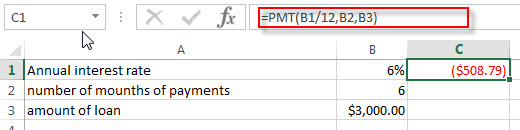### Related Functions

• Excel FV Function
The Excel FV function used to calculate the future value of an investment based on a constant interest rate. The syntax of the FV function is as below:=FV(rate,nper,pmt,[pv],[type])…
• Excel IPMT Function
The Excel IPMT function used to calculate the interest payment for an investment based on a constant payment schedule and a constant interest rage.The syntax of the IPMT function is as below:= IPMT (rate, per, nper, pv, [fv], [type])…
• Excel IRR Function
The Excel IRR function returns the internal rate of return for a series of cash flows and the cash flows must be occurred at regular intervals (monthly or annually).The syntax of the IRR function is as below:=IRR(values, [guess])…
• Excel ISPMT Function
The Excel ISPMT function used to calculate the interest paid during a specific period of an investment.The syntax of the ISPMT function is as below:= ISPMT (rate, per, nper, pv)…
• Excel MIRR Function
The Excel MIRR function returns the modified internal rate of return for a series of cash flows and the cash flows must be occurred at regular intervals (monthly or annually). The syntax of the MIRR function is as below:=MIRR(values, finance_rate,reinvest_rate)…
Excel NPER Function
The Excel NPER function returns the number of periods for an investment or loan based on periodic payment amount and a constant interest rate.The syntax of the NPER function is as below:= NPER (rate, pmt, pv, [fv], [type])…
• Excel NPV Function
The Excel NPV function returns the net present value of an investment by using a discount rate and a series of future cash flows (future payments and income).The syntax of the NPV function is as below:= NPV (rate, Value1,[value2],…)…
• Excel PPMT Function
The Excel PPMT function returns the payment amount on the principal for a given period for a loan or investment based on constant payments and a constant interest rate. The syntax of the PPMT function is as below:=PPMT(rate, per,nper, pv,[fv],[type])…
• Excel RATE Function
The Excel RATE function returns the interest rate per payment period of an annuity.The syntax of the RATE function is as below:=RATE(nper, pmt,pv,[fv],[type],[guess])…
• Excel PV Function
The Excel PV function returns the present value of a loan or investment based on constant payments and a constant interest rate. So you can use the PV function to get the present value based on a series of future payments.The syntax of the PV function is as below:= PV(rate,nper,pmt,[fv],[type])…

## Excel NPV Function

This post will guide you how to use Excel NPV function with syntax and examples in Microsoft excel.

### Description

The Excel NPV function returns the net present value of an investment by using a discount rate and a series of future cash flows (future payments and income).

The NPV function is a build-in function in Microsoft Excel and it is categorized as a Financial Function.

The NPV function is available in Excel 2016, Excel 2013, Excel 2010, Excel 2007, Excel 2011 for Mac.

### Syntax

The syntax of the NPV function is as below:

`= NPV (rate, Value1,[value2],…)`

Where the NPV function arguments are:

• Rate -This is a required argument. The discount rate over one period.
• Value1,[value2],… – the value1 argument is required, the subsequent values are optional. All values should be numeric values, and representing a series of future payments and income, the future payments should provide the negative values, and the income should provide the positive values.

Note:

• The NPV investment begins one period before the date of the value1 cash flow and ends with the last cash flow in the list. The NPV calculation is based on future cash flows. If your first cash flow occurs at the beginning of the first period, the first value must be added to the NPV result, not included in the values arguments. For more information, see the examples below.
• NPV is also related to the IRR function (internal rate of return). IRR is the rate for which NPV equals zero: NPV(IRR(…), …) = 0.

### Excel NPV Function Examples

The below examples will show you how to use Excel NPV Function to calculate the net present value of an investment using a discount rate and a series of future cash flows.

#1 to get the net present value of an investment, using the following formula:

`=NPV(B1,B2,B3,B4) `### Related Functions

• Excel FV Function
The Excel FV function used to calculate the future value of an investment based on a constant interest rate. The syntax of the FV function is as below:=FV(rate,nper,pmt,[pv],[type])…
• Excel IPMT Function
The Excel IPMT function used to calculate the interest payment for an investment based on a constant payment schedule and a constant interest rage.The syntax of the IPMT function is as below:= IPMT (rate, per, nper, pv, [fv], [type])…
• Excel IRR Function
The Excel IRR function returns the internal rate of return for a series of cash flows and the cash flows must be occurred at regular intervals (monthly or annually).The syntax of the IRR function is as below:=IRR(values, [guess])…
• Excel ISPMT Function
The Excel ISPMT function used to calculate the interest paid during a specific period of an investment.The syntax of the ISPMT function is as below:= ISPMT (rate, per, nper, pv)…
• Excel MIRR Function
The Excel MIRR function returns the modified internal rate of return for a series of cash flows and the cash flows must be occurred at regular intervals (monthly or annually). The syntax of the MIRR function is as below:=MIRR(values, finance_rate,reinvest_rate)…
Excel NPER Function
The Excel NPER function returns the number of periods for an investment or loan based on periodic payment amount and a constant interest rate.The syntax of the NPER function is as below:= NPER (rate, pmt, pv, [fv], [type])…
• Excel PMT Function
The Excel PMT function returns the payment amount for a loan or investment based on constant payments and a constant interest rate.The syntax of the PMT function is as below:= PMT(rate, nper, pv,[fv],[type])…
• Excel PPMT Function
The Excel PPMT function returns the payment amount on the principal for a given period for a loan or investment based on constant payments and a constant interest rate. The syntax of the PPMT function is as below:=PPMT(rate, per,nper, pv,[fv],[type])…
• Excel RATE Function
The Excel RATE function returns the interest rate per payment period of an annuity.The syntax of the RATE function is as below:=RATE(nper, pmt,pv,[fv],[type],[guess])…
• Excel PV Function
The Excel PV function returns the present value of a loan or investment based on constant payments and a constant interest rate. So you can use the PV function to get the present value based on a series of future payments.The syntax of the PV function is as below:= PV(rate,nper,pmt,[fv],[type])…

## Excel NPER Function

This post will guide you how to use Excel NPER function with syntax and examples in Microsoft excel.

### Description

The Excel NPER function returns the number of periods for an investment or loan based on periodic payment amount and a constant interest rate.

The NPER function is a build-in function in Microsoft Excel and it is categorized as a Financial Function.

The NPER function is available in Excel 2016, Excel 2013, Excel 2010, Excel 2007, Excel 2011 for Mac.

### Syntax

The syntax of the NPER function is as below:

`= NPER (rate, pmt, pv, [fv], [type])`

Where the NPER function arguments are:

• Rate -This is a required argument. The interest rate per period.
• Pmt– This is a required argument. The amount of the payment made each period. And if the Pmt argument is omitted, it will set as the default value 0.
• Pv – This is an optional argument. The present value of the payments. And if the PV argument is omitted, it will be set the default value as 0.
• FV– This is an optional argument. The Future value of the loan/investment at the end of nper payments. If it is omitted, it will be set the default value as 0.
• Type – This is an optional argument. It indicates when the payments are due. And if the type argument is omitted, it will be set as 0.  And the Type argument can have two value 0 or 1.
 Set type equal to If payments are due 0 At the end of the period 1 At the beginning of the period

### Excel NPER Function Examples

The below examples will show you how to use Excel NPER Function to get the number of payment periods for a loan.

#1 to get the periods for the investment, using the following formula:

`=NPER(B1/12,B2,B3,B4,B5)`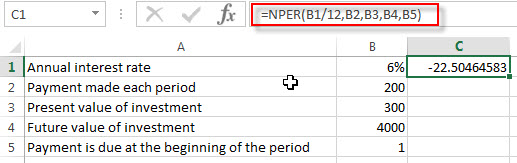### Related Functions

• Excel FV Function
The Excel FV function used to calculate the future value of an investment based on a constant interest rate. The syntax of the FV function is as below:=FV(rate,nper,pmt,[pv],[type])…
• Excel IPMT Function
The Excel IPMT function used to calculate the interest payment for an investment based on a constant payment schedule and a constant interest rage.The syntax of the IPMT function is as below:= IPMT (rate, per, nper, pv, [fv], [type])…
• Excel IRR Function
The Excel IRR function returns the internal rate of return for a series of cash flows and the cash flows must be occurred at regular intervals (monthly or annually).The syntax of the IRR function is as below:=IRR(values, [guess])…
• Excel ISPMT Function
The Excel ISPMT function used to calculate the interest paid during a specific period of an investment.The syntax of the ISPMT function is as below:= ISPMT (rate, per, nper, pv)…
• Excel MIRR Function
The Excel MIRR function returns the modified internal rate of return for a series of cash flows and the cash flows must be occurred at regular intervals (monthly or annually). The syntax of the MIRR function is as below:=MIRR(values, finance_rate,reinvest_rate)…
• Excel NPV Function
The Excel NPV function returns the net present value of an investment by using a discount rate and a series of future cash flows (future payments and income).The syntax of the NPV function is as below:= NPV (rate, Value1,[value2],…)…
• Excel PMT Function
The Excel PMT function returns the payment amount for a loan or investment based on constant payments and a constant interest rate.The syntax of the PMT function is as below:= PMT(rate, nper, pv,[fv],[type])…
• Excel PPMT Function
The Excel PPMT function returns the payment amount on the principal for a given period for a loan or investment based on constant payments and a constant interest rate. The syntax of the PPMT function is as below:=PPMT(rate, per,nper, pv,[fv],[type])…
• Excel RATE Function
The Excel RATE function returns the interest rate per payment period of an annuity.The syntax of the RATE function is as below:=RATE(nper, pmt,pv,[fv],[type],[guess])…
• Excel PV Function
The Excel PV function returns the present value of a loan or investment based on constant payments and a constant interest rate. So you can use the PV function to get the present value based on a series of future payments.The syntax of the PV function is as below:= PV(rate,nper,pmt,[fv],[type])…

## Excel MIRR Function

This post will guide you how to use Excel MIRR function with syntax and examples in Microsoft excel.

### Description

The Excel MIRR function returns the modified internal rate of return for a series of cash flows and the cash flows must be occurred at regular intervals (monthly or annually). MIRR considers both the cost of the investment and the interest received on reinvestment of cash.

The internal rate of return is the interest rate received for an investment consisting of payments (negative values) and income (positive values) that occur at regular periods.

The MIRR function is a build-in function in Microsoft Excel and it is categorized as a Financial Function.

The MIRR function is available in Excel 2016, Excel 2013, Excel 2010, Excel 2007, Excel 2011 for Mac.

### Syntax

The syntax of the MIRR function is as below:

`=MIRR(values, finance_rate,reinvest_rate)`

Where the MIRR function arguments are:

• Values -This is a required argument. An array or cell reference that contain numbers for which you want to calculate the internal rate of return. And the values must include at least one positive value and one negative value.
• finance_rate -This is a required argument. The interest rate that you pay on the cash flow amounts.
• reinvest_rate -This is a required argument. The interest rate paid on the reinvested cash flows.

Excel MIRR Function Examples

The below examples will show you how to use Excel MIRR Function to calculate the modified internal rate of return for a series of cash flows.

#1 to get the modified rate of return after 3 years, using the following formula:

`= MIRR (B1:B4)`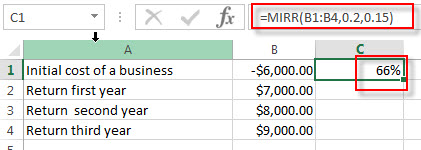### Related Functions

• Excel FV Function
The Excel FV function used to calculate the future value of an investment based on a constant interest rate. The syntax of the FV function is as below:=FV(rate,nper,pmt,[pv],[type])…
• Excel IPMT Function
The Excel IPMT function used to calculate the interest payment for an investment based on a constant payment schedule and a constant interest rage.The syntax of the IPMT function is as below:= IPMT (rate, per, nper, pv, [fv], [type])…
• Excel IRR Function
The Excel IRR function returns the internal rate of return for a series of cash flows and the cash flows must be occurred at regular intervals (monthly or annually).The syntax of the IRR function is as below:=IRR(values, [guess])…
• Excel ISPMT Function
The Excel ISPMT function used to calculate the interest paid during a specific period of an investment.The syntax of the ISPMT function is as below:= ISPMT (rate, per, nper, pv)…
• Excel NPER Function
The Excel NPER function returns the number of periods for an investment or loan based on periodic payment amount and a constant interest rate.The syntax of the NPER function is as below:= NPER (rate, pmt, pv, [fv], [type])…
• Excel NPV Function
The Excel NPV function returns the net present value of an investment by using a discount rate and a series of future cash flows (future payments and income).The syntax of the NPV function is as below:= NPV (rate, Value1,[value2],…)…
• Excel PMT Function
The Excel PMT function returns the payment amount for a loan or investment based on constant payments and a constant interest rate.The syntax of the PMT function is as below:= PMT(rate, nper, pv,[fv],[type])…
• Excel PPMT Function
The Excel PPMT function returns the payment amount on the principal for a given period for a loan or investment based on constant payments and a constant interest rate. The syntax of the PPMT function is as below:=PPMT(rate, per,nper, pv,[fv],[type])…
• Excel RATE Function
The Excel RATE function returns the interest rate per payment period of an annuity.The syntax of the RATE function is as below:=RATE(nper, pmt,pv,[fv],[type],[guess])…
• Excel PV Function
The Excel PV function returns the present value of a loan or investment based on constant payments and a constant interest rate. So you can use the PV function to get the present value based on a series of future payments.The syntax of the PV function is as below:= PV(rate,nper,pmt,[fv],[type])…

## Excel ISPMT Function

This post will guide you how to use Excel ISPMT function with syntax and examples in Microsoft excel.

### Description

The Excel ISPMT function used to calculate the interest paid during a specific period of an investment.

The ISPMT function is a build-in function in Microsoft Excel and it is categorized as a Financial Function.

The ISPMT function is available in Excel 2016, Excel 2013, Excel 2010, Excel 2007, Excel 2011 for Mac.

### Syntax

The syntax of the ISPMT function is as below:

`= ISPMT (rate, per, nper, pv)`

Where the ISPMT function arguments are:

• Rate -This is a required argument. The interest rate per period.
• Per –This is a required argument. The period for which you want to find the interest. And it must be an integer number between 1 and nper value.
• Nper – This is a required argument. The total number of payment periods for the annuity.
• Pv – This is a required argument. The present value of the payments.

Note:

• The units for rate and nper should be consistent. If you make monthly payments on a four-year loan at 12 percent annual interest, and you should use 12%/12 for rate and 4*12 for nper. If you make annual payments on the same loan, you need to use 12% for rate and 4 for nper.
• For all the arguments, cash you pay out, such as deposits to savings, is represented by negative numbers; cash you receive, such as dividend checks, is represented by positive numbers.

### Excel ISPMT Function Examples

The below examples will show you how to use Excel ISPMT Function to calculate the interest paid during a specific period of a loan or investment.

#1 to get the interest paid for the first year payment of a loan, using the following formula:

`= ISPMT(B1,B2,B3,B4)`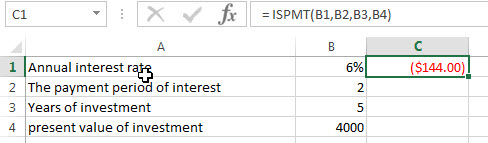### Related Functions

• Excel FV Function
The Excel FV function used to calculate the future value of an investment based on a constant interest rate. The syntax of the FV function is as below:=FV(rate,nper,pmt,[pv],[type])…
• Excel IPMT Function
The Excel IPMT function used to calculate the interest payment for an investment based on a constant payment schedule and a constant interest rage.The syntax of the IPMT function is as below:= IPMT (rate, per, nper, pv, [fv], [type])…
• Excel IRR Function
The Excel IRR function returns the internal rate of return for a series of cash flows and the cash flows must be occurred at regular intervals (monthly or annually).The syntax of the IRR function is as below:=IRR(values, [guess])…
• Excel MIRR Function
The Excel MIRR function returns the modified internal rate of return for a series of cash flows and the cash flows must be occurred at regular intervals (monthly or annually). The syntax of the MIRR function is as below:=MIRR(values, finance_rate,reinvest_rate)…
Excel NPER Function
The Excel NPER function returns the number of periods for an investment or loan based on periodic payment amount and a constant interest rate.The syntax of the NPER function is as below:= NPER (rate, pmt, pv, [fv], [type])…
• Excel NPV Function
The Excel NPV function returns the net present value of an investment by using a discount rate and a series of future cash flows (future payments and income).The syntax of the NPV function is as below:= NPV (rate, Value1,[value2],…)…
• Excel PMT Function
The Excel PMT function returns the payment amount for a loan or investment based on constant payments and a constant interest rate.The syntax of the PMT function is as below:= PMT(rate, nper, pv,[fv],[type])…
• Excel PPMT Function
The Excel PPMT function returns the payment amount on the principal for a given period for a loan or investment based on constant payments and a constant interest rate. The syntax of the PPMT function is as below:=PPMT(rate, per,nper, pv,[fv],[type])…
• Excel RATE Function
The Excel RATE function returns the interest rate per payment period of an annuity.The syntax of the RATE function is as below:=RATE(nper, pmt,pv,[fv],[type],[guess])…
• Excel PV Function
The Excel PV function returns the present value of a loan or investment based on constant payments and a constant interest rate. So you can use the PV function to get the present value based on a series of future payments.The syntax of the PV function is as below:= PV(rate,nper,pmt,[fv],[type])…

## Excel IRR Function

This post will guide you how to use Excel IRR function with syntax and examples in Microsoft excel.

### Description

The Excel IRR function returns the internal rate of return for a series of cash flows and the cash flows must be occurred at regular intervals (monthly or annually).

The internal rate of return is the interest rate received for an investment consisting of payments (negative values) and income (positive values) that occur at regular periods.

The IRR function is a build-in function in Microsoft Excel and it is categorized as a Financial Function.

The IRR function is available in Excel 2016, Excel 2013, Excel 2010, Excel 2007, Excel 2011 for Mac.

### Syntax

The syntax of the IRR function is as below:

`=IRR(values, [guess])`

Where the IRR function arguments are:

• Values -This is a required argument. An array or cell reference that contain numbers for which you want to calculate the internal rate of return. And the values must include at least one positive value and one negative value.
• Guess – This is an optional argument. An initial guess at the internal rate of return. If it is omitted, and it will be set as 0.1 or 10%.

Note:

• IRR is closely related to NPV, the net present value function. The rate of return calculated by IRR is the interest rate corresponding to a 0 (zero) net present value. The following formula demonstrates how NPV and IRR are related:
• NPV(IRR(A2:A7),A2:A7) equals 1.79E-09 [Within the accuracy of the IRR calculation, the value is effectively 0 (zero).]

### Excel IRR Function Examples

The below examples will show you how to use Excel IRR Function to calculate the internal rate of return for a series of cash flows.

#1 to get the investment’s internal rate of return after 3 years, using the following formula:

`=IRR(B1:B4)`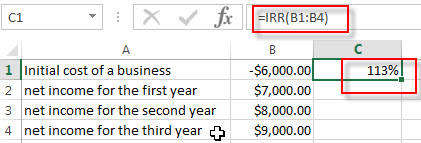### Related Functions

• Excel FV Function
The Excel FV function used to calculate the future value of an investment based on a constant interest rate. The syntax of the FV function is as below:=FV(rate,nper,pmt,[pv],[type])…
• Excel IPMT Function
The Excel IPMT function used to calculate the interest payment for an investment based on a constant payment schedule and a constant interest rage.The syntax of the IPMT function is as below:= IPMT (rate, per, nper, pv, [fv], [type])…
• Excel ISPMT Function
The Excel ISPMT function used to calculate the interest paid during a specific period of an investment.The syntax of the ISPMT function is as below:= ISPMT (rate, per, nper, pv)…
• Excel MIRR Function
The Excel MIRR function returns the modified internal rate of return for a series of cash flows and the cash flows must be occurred at regular intervals (monthly or annually). The syntax of the MIRR function is as below:=MIRR(values, finance_rate,reinvest_rate)…
Excel NPER Function
The Excel NPER function returns the number of periods for an investment or loan based on periodic payment amount and a constant interest rate.The syntax of the NPER function is as below:= NPER (rate, pmt, pv, [fv], [type])…
• Excel NPV Function
The Excel NPV function returns the net present value of an investment by using a discount rate and a series of future cash flows (future payments and income).The syntax of the NPV function is as below:= NPV (rate, Value1,[value2],…)…
• Excel PMT Function
The Excel PMT function returns the payment amount for a loan or investment based on constant payments and a constant interest rate.The syntax of the PMT function is as below:= PMT(rate, nper, pv,[fv],[type])…
• Excel PPMT Function
The Excel PPMT function returns the payment amount on the principal for a given period for a loan or investment based on constant payments and a constant interest rate. The syntax of the PPMT function is as below:=PPMT(rate, per,nper, pv,[fv],[type])…
• Excel RATE Function
The Excel RATE function returns the interest rate per payment period of an annuity.The syntax of the RATE function is as below:=RATE(nper, pmt,pv,[fv],[type],[guess])…
• Excel PV Function
The Excel PV function returns the present value of a loan or investment based on constant payments and a constant interest rate. So you can use the PV function to get the present value based on a series of future payments.The syntax of the PV function is as below:= PV(rate,nper,pmt,[fv],[type])…

## Excel IPMT Function

This post will guide you how to use Excel IPMT function with syntax and examples in Microsoft excel.

### Description

The Excel IPMT function used to calculate the interest payment for an investment based on a constant payment schedule and a constant interest rage.

The IPMT function is a build-in function in Microsoft Excel and it is categorized as a Financial Function.

The IPMT function is available in Excel 2016, Excel 2013, Excel 2010, Excel 2007, Excel 2011 for Mac.

### Syntax

The syntax of the IPMT function is as below:

`= IPMT (rate, per, nper, pv, [fv], [type])`

Where the IPMT function arguments are:

• Rate -This is a required argument. The interest rate per period.
• Per – -This is a required argument. The period for which you want to find the interest. And it must be an integer number between 1 and nper value.
• Nper– This is a required argument. The total number of payment periods for the annuity.
• Pv – This is a required argument. The present value of the payments.
• FV– This is an optional argument. The Future value of the loan/investment at the end of nper payments. If it is omitted, it will be set the default value as 0.
• Type – This is an optional argument. It indicates when the payments are due. And if the type argument is omitted, it will be set as 0.  And the Type argument can have two value 0 or 1.
 Set type equal to If payments are due 0 At the end of the period 1 At the beginning of the period

Note:

• The units for rate and nper should be consistent. If you make monthly payments on a four-year loan at 12 percent annual interest, and you should use 12%/12 for rate and 4*12 for nper. If you make annual payments on the same loan, you need to use 12% for rate and 4 for nper.
• For all the arguments, cash you pay out, such as deposits to savings, is represented by negative numbers; cash you receive, such as dividend checks, is represented by positive numbers.

### Excel IPMT Function Examples

The below examples will show you how to use Excel IPMT Function to calculate the interest payment based on a specific period of an investment with a constant interest rage.

#1 to get the interest payment due in the first mount for an investment, using the following formula:

`= IPMT(B1/12,B2,B3*12,B4,B5)`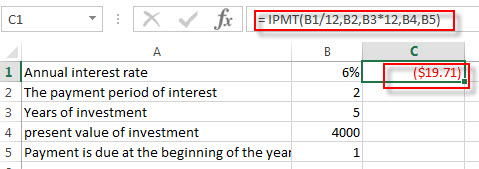### Related Functions

• Excel FV Function
The Excel FV function used to calculate the future value of an investment based on a constant interest rate. The syntax of the FV function is as below:=FV(rate,nper,pmt,[pv],[type])…
• Excel IRR Function
The Excel IRR function returns the internal rate of return for a series of cash flows and the cash flows must be occurred at regular intervals (monthly or annually).The syntax of the IRR function is as below:=IRR(values, [guess])…
• Excel ISPMT Function
The Excel ISPMT function used to calculate the interest paid during a specific period of an investment.The syntax of the ISPMT function is as below:= ISPMT (rate, per, nper, pv)…
• Excel MIRR Function
The Excel MIRR function returns the modified internal rate of return for a series of cash flows and the cash flows must be occurred at regular intervals (monthly or annually). The syntax of the MIRR function is as below:=MIRR(values, finance_rate,reinvest_rate)…
Excel NPER Function
The Excel NPER function returns the number of periods for an investment or loan based on periodic payment amount and a constant interest rate.The syntax of the NPER function is as below:= NPER (rate, pmt, pv, [fv], [type])…
• Excel NPV Function
The Excel NPV function returns the net present value of an investment by using a discount rate and a series of future cash flows (future payments and income).The syntax of the NPV function is as below:= NPV (rate, Value1,[value2],…)…
• Excel PMT Function
The Excel PMT function returns the payment amount for a loan or investment based on constant payments and a constant interest rate.The syntax of the PMT function is as below:= PMT(rate, nper, pv,[fv],[type])…
• Excel PPMT Function
The Excel PPMT function returns the payment amount on the principal for a given period for a loan or investment based on constant payments and a constant interest rate. The syntax of the PPMT function is as below:=PPMT(rate, per,nper, pv,[fv],[type])…
• Excel RATE Function
The Excel RATE function returns the interest rate per payment period of an annuity.The syntax of the RATE function is as below:=RATE(nper, pmt,pv,[fv],[type],[guess])…
• Excel PV Function
The Excel PV function returns the present value of a loan or investment based on constant payments and a constant interest rate. So you can use the PV function to get the present value based on a series of future payments.The syntax of the PV function is as below:= PV(rate,nper,pmt,[fv],[type])…

## Excel FV Function

This post will guide you how to use Excel FV function with syntax and examples in Microsoft excel.

### Description

The Excel FV function used to calculate the future value of an investment based on a constant interest rate. So you can use the FV function to get the future value of an investment with either periodic, constant payments.

The FV function is a build-in function in Microsoft Excel and it is categorized as a Financial Function.

The FV function is available in Excel 2016, Excel 2013, Excel 2010, Excel 2007, Excel 2011 for Mac.

### Syntax

The syntax of the FV function is as below:

`=FV(rate,nper,pmt,[pv],[type])`

Where the FV function arguments are:

• Rate -This is a required argument. The interest rate per period.
• Nper– This is a required argument. The total number of payment periods for the annuity.
• Pmt– This is a required argument. The amount of the payment made each period. And if the Pmt argument is omitted, it will set as the default value 0.
• Pv – This is an optional argument. The present value of the payments. And if the PV argument is omitted, it will be set the default value as 0.
• Type – This is an optional argument. It indicates when the payments are due. And if the type argument is omitted, it will be set as 0.  And the Type argument can have two value 0 or 1.
 Set type equal to If payments are due 0 At the end of the period 1 At the beginning of the period

Note:

• The units for rate and nper should be consistent. If you make monthly payments on a four-year loan at 12 percent annual interest, and you should use 12%/12 for rate and 4*12 for nper. If you make annual payments on the same loan, you need to use 12% for rate and 4 for nper.
• For all the arguments, cash you pay out, such as deposits to savings, is represented by negative numbers; cash you receive, such as dividend checks, is represented by positive numbers.

### Excel FV Function Examples

The below examples will show you how to use Excel FV Function to calculate the future value of an investment with a constant interest rate.

#1 to get the future value of an investment, using the following formula:

`= FV (B1/12,B2,B3,B4,B5)`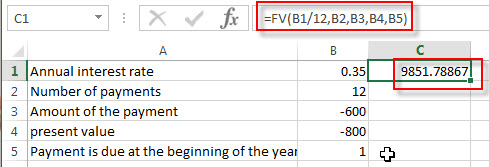### Related Functions

• Excel IPMT Function
The Excel IPMT function used to calculate the interest payment for an investment based on a constant payment schedule and a constant interest rage.The syntax of the IPMT function is as below:= IPMT (rate, per, nper, pv, [fv], [type])…
• Excel IRR Function
The Excel IRR function returns the internal rate of return for a series of cash flows and the cash flows must be occurred at regular intervals (monthly or annually).The syntax of the IRR function is as below:=IRR(values, [guess])…
• Excel ISPMT Function
The Excel ISPMT function used to calculate the interest paid during a specific period of an investment.The syntax of the ISPMT function is as below:= ISPMT (rate, per, nper, pv)…
• Excel MIRR Function
The Excel MIRR function returns the modified internal rate of return for a series of cash flows and the cash flows must be occurred at regular intervals (monthly or annually). The syntax of the MIRR function is as below:=MIRR(values, finance_rate,reinvest_rate)…
Excel NPER Function
The Excel NPER function returns the number of periods for an investment or loan based on periodic payment amount and a constant interest rate.The syntax of the NPER function is as below:= NPER (rate, pmt, pv, [fv], [type])…
• Excel NPV Function
The Excel NPV function returns the net present value of an investment by using a discount rate and a series of future cash flows (future payments and income).The syntax of the NPV function is as below:= NPV (rate, Value1,[value2],…)…
• Excel PMT Function
The Excel PMT function returns the payment amount for a loan or investment based on constant payments and a constant interest rate.The syntax of the PMT function is as below:= PMT(rate, nper, pv,[fv],[type])…
• Excel PPMT Function
The Excel PPMT function returns the payment amount on the principal for a given period for a loan or investment based on constant payments and a constant interest rate. The syntax of the PPMT function is as below:=PPMT(rate, per,nper, pv,[fv],[type])…
• Excel RATE Function
The Excel RATE function returns the interest rate per payment period of an annuity.The syntax of the RATE function is as below:=RATE(nper, pmt,pv,[fv],[type],[guess])…
• Excel PV Function
The Excel PV function returns the present value of a loan or investment based on constant payments and a constant interest rate. So you can use the PV function to get the present value based on a series of future payments.The syntax of the PV function is as below:= PV(rate,nper,pmt,[fv],[type])…

## Excel DDB Function

This post will guide you how to use Excel DDB function with syntax and examples in Microsoft excel.

### Description

The Excel DB function returns the depreciation of an asset for a specified period using the double-declining balance method or you can also use some other method to get the depreciation.

The DB function is a build-in function in Microsoft Excel and it is categorized as a Financial Function.

The DB function is available in Excel 2016, Excel 2013, Excel 2010, Excel 2007, Excel 2011 for Mac.

### Syntax

The syntax of the DDB function is as below:

`= DDB(cost, salvage, life, period, [factor])`

Where the DDB function arguments are:

• Cost -This is a required argument. The original cost of the asset.
• Salvage – This is a required argument.  The salvage value at the end of the life of the asset.
• Life – This is a required argument.  The number of periods over which the asset is to be depreciated.
• Period – This is a required argument.  The period in which to calculate the depreciation.
• Factor – This is an optional argument.  The rate at which the balance declines. If the factor argument is omitted, and it will be set the default value as 2.

Note:

• If you do not use the double declining balance method, you can change the factor argument to other value.
• You can use the VDB function if you want to switch to the straight-line depreciation method when depreciation is greater than the declining balance calculation.

### Excel DDB Function Examples

The below examples will show you how to use Excel DB Function to calculate the depreciation of an asset using the double declining balance method.

#1 to get the second day’s depreciation using the double declining balance method, refer to the following formula:

`=DDB(B1,B2,B3,1)`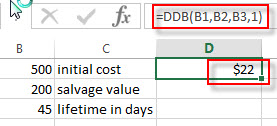#2 to get the month’s depreciation using the double declining balance method, enter the following formula in Cell C2:

`=DDB(B1,B2,B3*12,1)`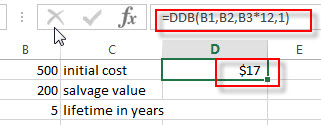### Related Functions

• Excel DB Function
The Excel DB function returns the depreciation of an asset for a specified period using the fixed-declining balance method.The syntax of the DB function is as below:= DB (cost, salvage, life, period, [month])…

## Excel DB Function

This post will guide you how to use Excel DB function with syntax and examples in Microsoft excel.

### Description

The Excel DB function returns the depreciation of an asset for a specified period using the fixed-declining balance method.

The DB function is a build-in function in Microsoft Excel and it is categorized as a Financial Function.

The DB function is available in Excel 2016, Excel 2013, Excel 2010, Excel 2007, Excel 2011 for Mac.

### Syntax

The syntax of the DB function is as below:

`= DB (cost, salvage, life, period, [month])`

Where the DB function arguments are:

• Cost -This is a required argument. The original cost of the asset.
• Salvage – This is a required argument.  The salvage value at the end of the life of the asset.
• Life – This is a required argument.  The number of periods over which the asset is to be depreciated.
• Period – This is a required argument.  The period in which to calculate the depreciation.
• Month – This is an optional argument.  The number of months in the first year of depreciation. The default value is 12.

### Excel DB Function Examples

The below examples will show you how to use Excel DB Function to calculate the depreciation of an asset using the fixed declining balance method.

#1 =DB(B1,B2,B3,B4,B5)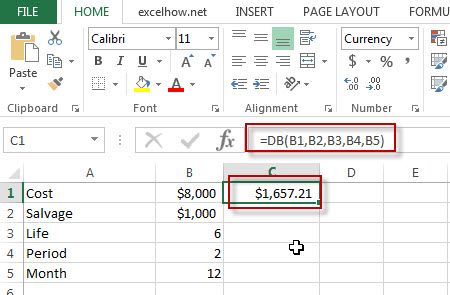### Related Functions

• Excel DDB Function
The Excel DB function returns the depreciation of an asset for a specified period using the double-declining balance method or you can also use some other method to get the depreciation.The syntax of the DDB function is as below:= DDB(cost, salvage, life, period, [factor])…

## Excel Amorlinc Function

This post will guide you how to use Excel AMORLINC function with syntax and examples in Microsoft excel.

### Description

The Excel AMORLINC function returns the linear depreciation of an asset for each accounting period. This function is provided for French accounting system.

The AMORLINC function is a build-in function in Microsoft Excel and it is categorized as a Financial Function.

The AMORLINC function is available in Excel 2016, Excel 2013, Excel 2010, Excel 2007, Excel 2011 for Mac.

### Syntax

The syntax of the AMORLINC function is as below:

`= AMORLINC(cost, date_purchased, first_period, salvage, period, rate, [basis])`

Where the AMORLINC function arguments are:

• Cost -This is a required argument. The cost of the asset.
• Date_purchased – This is a required argument.  The date that the asset was purchased.
• First_period – This is a required argument.  The date of the end of the first period.
• Salvage – This is a required argument.  The salvage value at the end of the life of the asset.
• Period – This is a required argument.  The period in which to calculate the depreciation.
• Rate– This is a required argument.  The rate of depreciation.
• Basis – This is a required argument.  The year basis to be used. The following values can be used:
 Basis Date system 0 or omitted 360 days (NASD method) 1 Actual 3 365 days in a year 4 360 days in a year (European method)

### Excel AMORLINC  Function Example

The below examples will show you how to use Excel AMORLINC Function to calculate the linear depreciation of an asset for each accounting period.

#1 = AMORLINC (B1,B2,B3,B4,B5,B6,B7)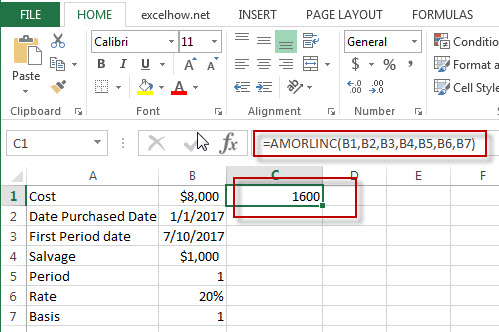### Related Functions

• Excel Amordegrc Function
The Excel AMORDEGRC function returns the linear depreciation of an asset for each accounting period by using a depreciation coefficient.The syntax of the AMORDEGRC function is as below:= AMORDEGRC (cost, date_purchased, first_period, salvage, period, rate, [basis])…

## Excel Amordegrc Function

This post will guide you how to use Excel AMORDEGRC function with syntax and examples in Microsoft excel.

### Description

The Excel AMORDEGRC function returns the linear depreciation of an asset for each accounting period by using a depreciation coefficient.

The AMORDEGRC function is a build-in function in Microsoft Excel and it is categorized as a Financial Function.

The AMORDEGRC function is available in Excel 2016, Excel 2013, Excel 2010, Excel 2007, Excel 2011 for Mac.

### Syntax

The syntax of the AMORDEGRC function is as below:

`= AMORDEGRC (cost, date_purchased, first_period, salvage, period, rate, [basis])`

Where the AMORDEGRC function arguments are:

• Cost -This is a required argument. The cost of the asset.
• Date_purchased – This is a required argument.  The date that the asset was purchased.
• First_period – This is a required argument.  The date of the end of the first period.
• Salvage – This is a required argument.  The salvage value at the end of the life of the asset.
• Period – This is a required argument.  The period in which to calculate the depreciation.
• Rate– This is a required argument.  The rate of depreciation.
• Basis – This is a required argument.  The year basis to be used. The following values can be used:
 Basis Date system 0 or omitted 360 days (NASD method) 1 Actual 3 365 days in a year 4 360 days in a year (European method)

### Excel AMORDEGRC Function Examples

The below examples will show you how to use Excel AMORDEGRC Function to calculate the linear depreciation of an asset for each accounting period.

#1 =AMORDEGRC(B1,B2,B3,B4,B5,B6,B7)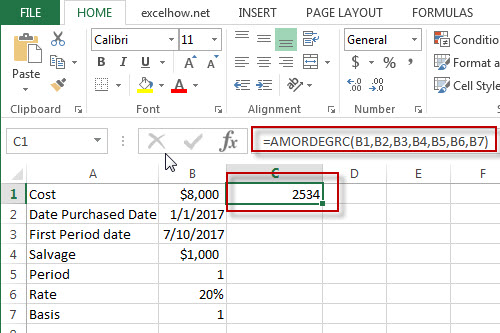### Related Functions

• Excel Amorlinc Function
The Excel AMORLINC function returns the linear depreciation of an asset for each accounting period. This function is provided for French accounting system.The syntax of the AMORLINC function is as below:= AMORLINC (cost, date_purchased, first_period, salvage, period, rate, [basis])…

## Excel AccrintM Function

This post will guide you how to use Excel ACCRINTM function with syntax and examples in Microsoft excel.

### Description

The Excel ACCRINTM function returns the accrued interest for a security that pays interest at maturity.

The ACCRINTM function is a build-in function in Microsoft Excel and it is categorized as a Financial Function.

The ACCRINTM function is available in Excel 2016, Excel 2013, Excel 2010, Excel 2007,  Excel 2011 for Mac.

### Syntax

The syntax of the ACCRINTM function is as below:

`= ACCRINTM(issue, settlement, rate, par, [basis])`

Where the ACCRINTM function arguments are:

• Issue -This is a required argument. The security’s issue date.
• Sattlement – This is a required argument.  The security’s settlement date.
• Rate – This is a required argument.  The security’s annual coupon rate.
• Par – This is a required argument.  The par value of the security. Note: If Par is omitted, the default Par value will be set to \$1000.
• Basis – This is an optional argument. The type of day count basis to use.  it can be set as the following values:
 Basis Day count basis 0 or omitted US (NASD) 30/360 1 Actual/actual 2 Actual/360 3 Actual/365 4 European 30/360

### Excel ACCRINTM Function Example

The below examples will show you how to use Excel ACCRINTM Function to return the accrued interest for a security that pays interest at maturity.

`#1 =ACCRINTM(B1,B2,B3,B4,B5)`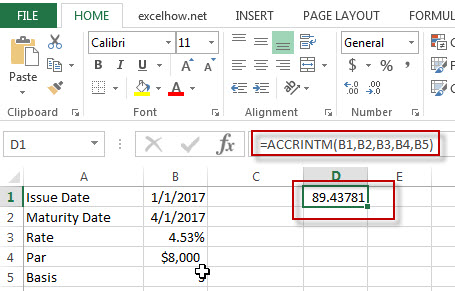### Related Functions

• Excel Accrint Function
The Excel ACCRINT function returns the accrued interest for a security that pays periodic interest.The syntax of the ACCRINT function is as below:= ACCRINT (issue, first_interest, settlement, rate, par, frequency, [basis], [calc_method])